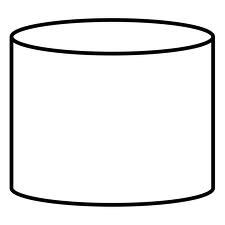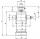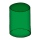# Cylinder twice

If the radius of the cylinder increases twice, and the height is reduced twice, then the volume of the cylinder increases (how many times?):

n =  2

### Step-by-step explanation:Did you find an error or inaccuracy? Feel free to write us. Thank you!Tips to related online calculators
Tip: Our volume units converter will help you with the conversion of volume units.

## Related math problems and questions:

• Volume of sphereHow many times does the volume of a sphere increase if its radius increases 2 ×?How many times does the surface of a sphere decrease if we reduce its radius twice?
• Cylinder - hThe cylinder volume is 140 cm3. The base radius is 7 cm. Calculate the height of the cylinder.
• Perimeter of baseThe circumference of the base of the rotating cylinder is same as its height. What is the diameter and height of this cylinder with volume 1 liter?
• BottleA company wants to produce a bottle whose capacity is 1.25 liters. Find the dimensions of a cylinder that will be required to produce this 1.25litres if the hight of the cylinder must be 5 times the radius.
• Cylinder and its circumferenceIf the height of a cylinder is 4 times its circumference c, what is the volume of the cylinder in terms of its circumference, c?
• DiameterWhat is the inside diameter of the cylinder container and if half a liter of water reaches a height 15 cm?
• The potThe pot is a cylinder with a volume of V = 7l and an inner diameter of d = 20cm. Find its depth.
• Cube into cylinderIf we dip a wooden cube into a barrel with a 40cm radius, the water will rise 10 cm. What is the size of the cube edge?
• Height of the cylinderThe cylinder volume is 150 dm cubic, the base diameter is 100 cm. What is the height of the cylinder?
• Cuboid enlargementBy how many percent increases the volume of cuboid if every dimension increases by 30%?
• CylinderThe cylinder surface is 922 dm2. Its height is equal to the radius of the base. Calculate the height of this cylinder.
• ScaleCylinder was drawn in scale 2:1. How many times is the volume of the cylinder smaller in reality?
• Water cylinderZuzana poured 785 ml of water into a measuring cylinder with a base radius of 5 cm. The water in the cylinder reached a height of 2 cm from the upper edge of the cylinder. How tall is the cylinder? (π = 3.14)
• CuboidHow many times will increase the volume of a cuboid, if one dimension is twice larger, second dimension three times larger and third dimension four times lower?
• Cylinder containerIf the cylinder-shaped container is filled with water to a height of 5 dm, it contains 62.8 hectoliters of water. Calculate the diameter of the bottom of the container. Use the value π = 3.14.
• Cylindrical tankCylindrical tank holds 600hl water and is deep 2.5 m. Calculate the diameter of the cylinder.